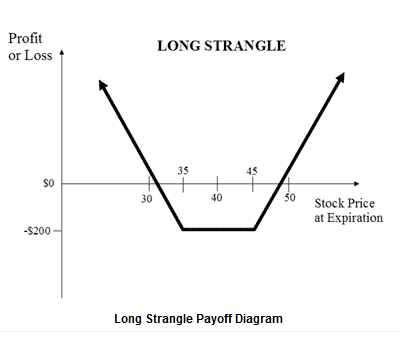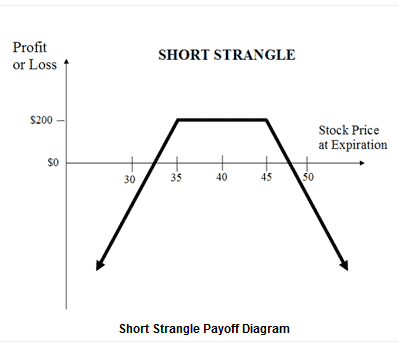• 价格总览
• 费用

交易策略

杠杆:无限盈利可能

• 最大利润 = 无限制
• 满足条件即可盈利： 标的股票价格 >= 行权价 + 期权金
• 盈利 = 标的股票价格 – 行权价 – 期权金

有限风险

• 最大亏损 = 期权金 + 佣金
• 当标的股票价格 <= 看涨期权行权价时，亏损最大

盈亏平衡点

• 盈亏平衡价 = 行权价 + 期权金

• 看跌期权熊市价差构建
• 买入 1 手价内看跌期权
• 卖出 1 手价外看跌期权有限下跌盈利

• 最大盈利 = 买入卖权行权价 – 卖出卖权行权价 – 净期权金 – 佣金
• 当标的股票价格 <= 卖出卖权行权价时，获得最大盈利

有限上涨风险

• 最大亏损 = 净期权金 + 佣金
• 当标的股票价格 >= 买入卖权行权价时，亏损最大

盈亏平衡点

• 盈亏平衡价 = 买入卖权行权价 – 净期权金

卖出 1 手价外看涨期权有限上涨盈利

• 最大盈利 = 卖出买权的行权价 – 买入买权的行权价 – 净期权金 – 佣金
• 当标的股票价格 >= 卖出买权行权价时，获得最大盈利

有限下跌风险

• 最大亏损 = 净期权金 + 佣金
• 当标的股票价格 <= 买入买权行权价时，亏损最大

盈亏平衡点

• 盈亏平衡价 = 买入买权行权价 + 净期权金

价外保护性买权有限盈利可能

• 最大盈利 = 期权金 – 标的股票购买价格 + 卖出买权行使价 – 佣金
• 当标的股票价格 >= 卖出买权行使价时，获得最大盈利

无限亏损可能

• 最大亏损 = 无限
• 当标的股票价格 < 标的股票购买价格 – 期权金时，发生亏损
• 亏损 = 标的股票购买价格 – 标的股票价格 – 最大盈利 + 佣金

盈亏平衡点

• 盈亏平衡价 = 标的股票购买价格 – 期权金有限盈亏可能

• 最大盈利 = 无限
• 当标的股票价格降至 0 时，盈利最大
• 盈利 = 买入卖权行权价 – 期权金

有限风险

• 最大亏损 = 期权金 + 佣金
• 当标的股票价格 >= 买入卖权行权价时，亏损最大

盈亏平衡点

• 盈亏平衡价 = 买入卖权行权价 – 期权金

买入 1 手价外看跌期权无限盈利可能

• 最大盈利无限
• 当标的股票价格 > 买入买权行权价 + 净期权金 或 标的股票价格 < 买入卖权行权价 – 净期权金时盈利
• 盈利 = 标的股票价格 – 买入买权行权价 – 净期权金 或 买入卖权行权价 – 标的股票价格 – 净期权金

有限风险

• 最大亏损 = 净期权金 + 佣金
• 标的股票价格在买入买权行使价和买入卖权行使价之间时产生最大亏损

盈亏平衡点

• 较高的盈亏平衡价 = 买入买权行权价 + 净权利金
• 较低的盈亏平衡价 = 买入卖权行权价 – 净权利金

The long strangle, is a neutral strategy in options trading that involves the simultaneous buying of a slightly out-of-the-money put and a slightly out-of-the-money call of the same underlying asset and expiration date.

Buy 1 OTM Put

The long options strangle is an unlimited profit/limited risk strategy used when the options trader thinks that the underlying stock will experience significant volatility in the near term. Long strangles are debit spreads as a net debit is taken to enter the trade.Unlimited profit potential

A large gain for the long straddle option strategy is attainable when the underlying stock price makes a very strong move either upwards or downwards at expiration.

The formula for calculating profit is given below:

• Maximum Profit Unlimited
• Profit Achieved When Price of Underlying > Strike Price of Long Call + Net Premium Paid or Price of Underlying < strike Price of Long Put – Net Premium Paid
• Profit = Price of Underlying – Strike Price of Long Call – Net Premium Paid or Strike Price of Long Put – Price of Underlying – Net Premium Paid

Limited risk

Maximum loss for the long strangle options strategy is hit when the underlying stock price on expiration date is trading between the strike prices of the options bought. At this price, both options expire worthless and the options trader loses the entire initial debit taken to enter the trade.

The formula for calculating maximum loss is given below:

• Max Loss = Net Premium Paid + Commissions Paid
• Max Loss Occurs When Price of Underlying is in between Strike Price of Long Call and Strike Price of Long Put

Breakeven points

There are two breakeven points for the long strangle position. The breakeven points can be calculated using the following formulae:

• Upper Breakeven Point = Strike Price of Long Call + Net Premium Paid
• Lower Breakeven Point = Strike Price of Long Put – Net Premium Paid有限盈利可能

• 最大盈利 = 收到的权利金 – 佣金支付
• 当标的股票价格 <= 卖出买权行权价时，获得最大盈利

无限亏损风险

• 最大亏损 = 无限
• 当标的股票价格 > 卖出买权行权价 + 权利金时，产生亏损
• 亏损 = 标的股票价格 – 卖出买权行权价 – 收到的权利金 + 佣金支付

盈亏平衡点

• 盈亏平衡价 = 卖出买权行权价 + 权利金

有限盈利可能• 最大盈利 = 卖出买权行权价 – 标的股票购买价格 + 净期权金收入 – 佣金支付
• 当标的股票价格 >= 卖出买权行权价时，获得最大盈利

有限风险

• 最大亏损 = 标的股票购买价格 – 买入卖权行权价 – 净权利金收入 + 佣金支付
• 当标的股票价格 <= 买入卖权行权价

盈亏平衡点

• 盈亏平衡价 = 标的股票购买价格 + 净权利金支付

卖出 1 手平价看跌期权有限盈利，无上涨风险

• 最大盈利 = 期权金收入 - 佣金支付
• 当标的股票价格 >= 卖出卖权行权价时，获得最大盈利

无限下跌风险，几乎无下跌保护

• 最大亏损 = 无限
• 当标的股票价格 < 卖出卖权行权价 – 期权金收入时，产生亏损
• 亏损 = 卖出卖权行权价 – 标的股票价格 – 期权金收入 + 佣金支付

盈亏平衡点

• 盈亏平衡价 = 卖出卖权行权价 – 期权金收入

卖出 1 手平价看跌期权有限盈利

• 最大盈利 = 净期权金收入
• 当标的股票价格 = 卖出看涨/看跌期权行权价时，盈利最大化

无限风险

• 最大亏损 = 无限
• 当标的股票价格 > 卖出买权行权价 + 净权利金收入 or 标的股票价格 < 卖出卖权行权价 – 净权利金收入时，产生亏损

盈亏平衡点

• 较高的盈亏平衡价 = 卖出买权行权价 + 净权利金收入
• 较低的盈亏平衡价 = 卖出卖权行权价 – 净权利金收入

The short strangle option strategy is a limited profit/unlimited risk options trading strategy that is used when the options trader thinks that the underlying stock will experience little volatility in the near term.

Sell 1 OTM PutLimited profit

Maximum profit for the short strangle occurs when the underlying stock price on expiration date is trading between the strike prices of the options sold. At this price, both options expire worthless and the options trader gets to keep the entire initial credit taken as profit.

The formula for calculating maximum profit is given below:

• Max Profit = Net Premium Received
• Max Profit Achieved When Price of underlying is inbetween the Strike Price of the Short Call and the Strike Price of the Short Put

Unlimited risk

Large losses for the short strangle can be experienced when the underlying stock price makes a strong move either upwards or downwards at expiration.

The formula for calculating loss is given below:

• Maximum Loss = Unlimited
• Loss Occurs When Price of underlying > Strike Price of short Call + Net Premium Received or Price of Underlying < Strike Price of Short Put – Net Premium Received.
• Loss = Price of Underlying – strike Price of Short Call – Net Premium received or Strike Price of short Put – Price of Underlying – Net Premium Received.

Breakeven points

There are two breakeven points for the short strangle position. The breakeven points can be calculated using the following formulae:

• Upper Breakeven Point = Strike Price of Short Call + Net Premium Received
• Lower Breakeven Point = Strike Price of Short Put – Net Premium Received

股票期权风险警告期权被归类为红色产品，因为它被视为高度复杂而且高风险的投资产品。中文Apple, iPad 和 iPhone是苹果公司在美国和其他国家注册的商标。App Store是苹果公司的服务商标。